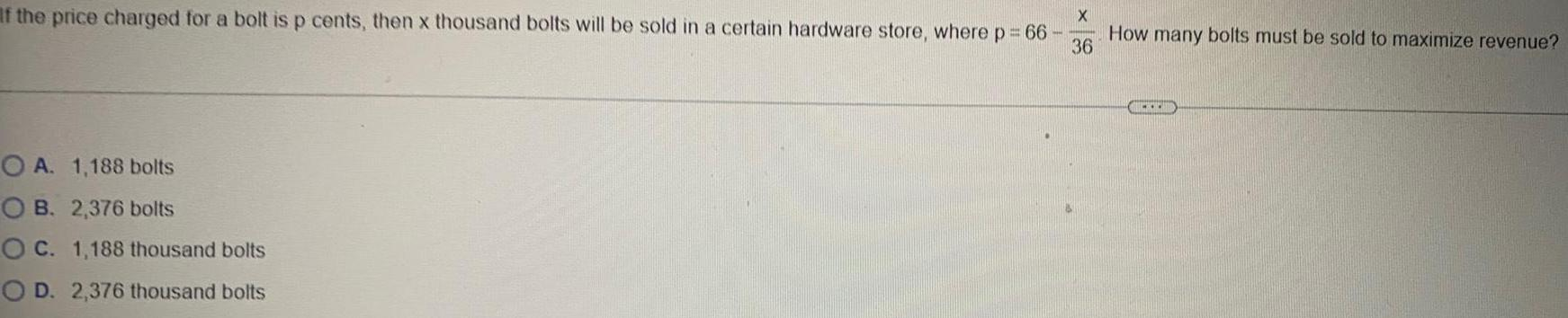Math
Differentiation
If the price charged for a bolt is p cents, then x thousand bolts will be sold in a certain hardware store, where p = 66- x/36. How many bolts must be sold to maximize revenue? A. 1,188 bolts B. 2,376 bolts C. 1,188 thousand bolts D. 2,376 thousand bolts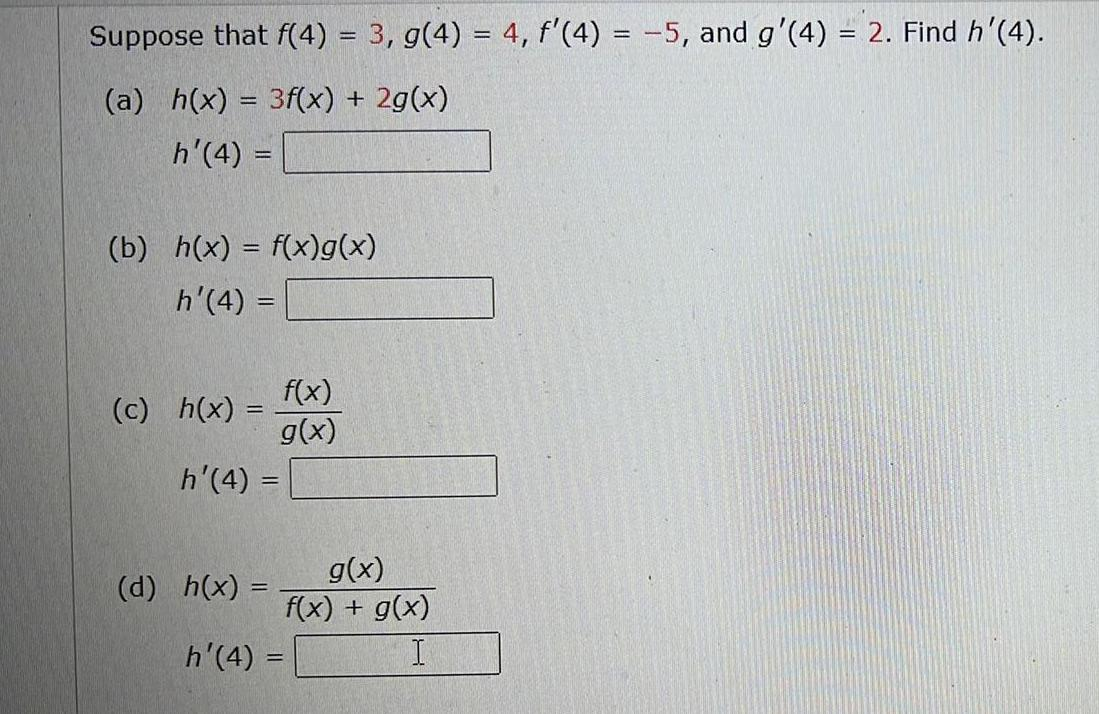Math
Differentiation
Suppose that f(4) = 3, g(4) = 4, f'(4) = -5, and g'(4) = 2. Find h'(4). (a) h(x) = 3f(x) + 2g(x) h'(4) = (b) h(x) = f(x)g(x) h'(4) = (c) h(x) =f(x)/g(x) h'(4) = (d) h(x) =(g(x))/(f(x) + g(x)) h'(4) =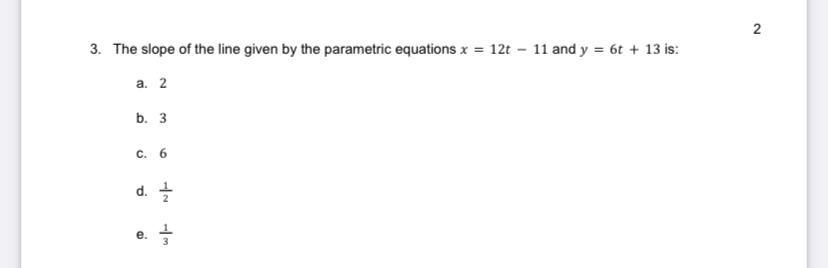Calculus
Differentiation
The slope of the line given by the parametric equations x =12t-11 and y = 6t+13 is: a. 2 b.3 c. 6 d. 1/2 e.1/3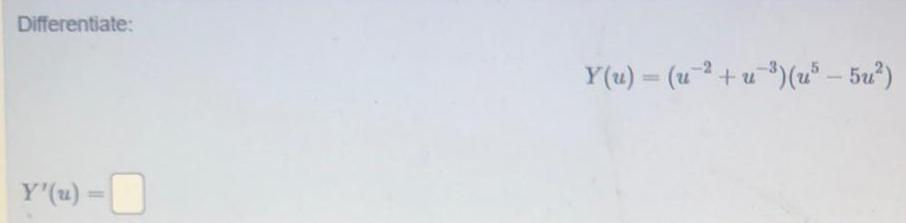Math
Differentiation
Differentiate: Y'(u) = Y(u) = (u2+u-³) (u³ - 5u²)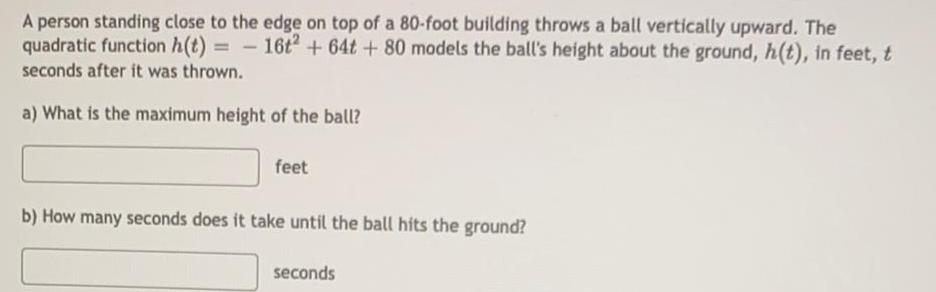Math
Differentiation
A person standing close to the edge on top of a 80-foot building throws a ball vertically upward. The quadratic function h(t) 16t^,2 +64t+ 80 models the ball's height about the ground, h(t), in feet, t seconds after it was thrown. a) What is the maximum height of the ball? feet b) How many seconds does it take until the ball hits the ground? seconds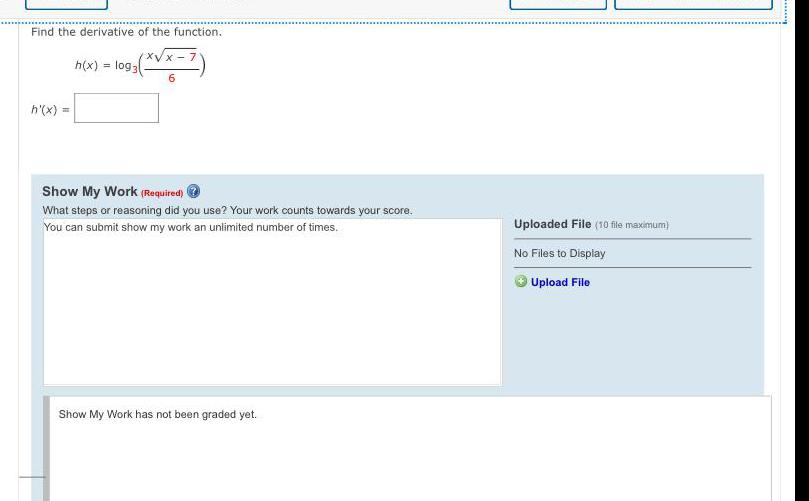Math
Differentiation
Find the derivative of the function. h(x) = log3 x√x - 7/6 h'(x)=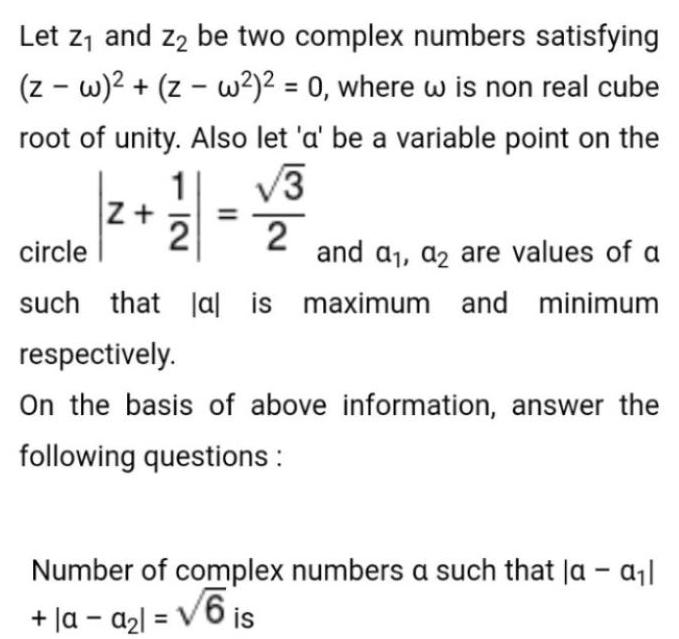Math
Differentiation
Let z₁ and z₂ be two complex numbers satisfying (z-w)²+(z-w²)² = 0, where w is non real cube root of unity. Also let 'a' be a variable point on the circle |z+1/2|=√3/2 and a₁, a2 are values of a such that lal is maximum and minimum respectively. On the basis of above information, answer the following questions : Number of complex numbers a such that la - a₁l +|a -a₂l= √6 is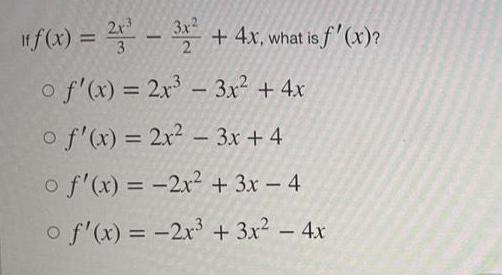Math
Differentiation
If f(x) = 2x3/3 - 3x²/2 + 4x, what is f'(x)? f'(x) = 2x³ - 3x² + 4x f'(x) = 2x² - 3x + 4 f'(x) = -2x² + 3x - 4 f'(x) = -2x³ + 3x² - 4x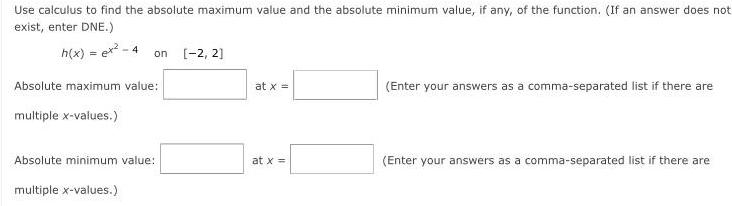Math
Differentiation
Use calculus to find the absolute maximum value and the absolute minimum value, if any, of the function. (If an answer does not exist, enter DNE.) h(x) = ex² - 4 on [-2, 2] Absolute maximum value: at x = (Enter your answers as a comma-separated list if there are multiple x-values.) Absolute minimum value: at x = (Enter your answers as a comma-separated list if there are multiple x-values.)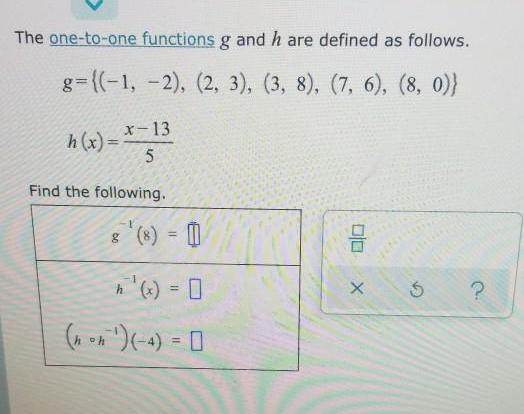Math
Differentiation
The one-to-one functions g and h are defined as follows. g={(-1, -2), (2, 3), (3, 8), (7, 6), (8, 0)) h(x)= x-13/5 Find the following. g^-1(8)= h^-1(x) (hoh^-1)(-4)=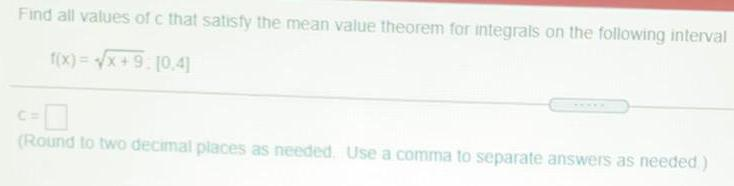Math
Differentiation
Find all values of c that satisfy the mean value theorem for integrals on the following interval f(x)=√x+9 . [0.4]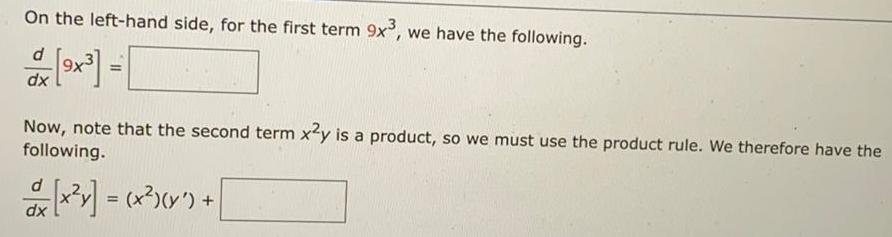Math
Differentiation
On the left-hand side, for the first term 9x³, we have the following. d/dx[9x³]=_ Now, note that the second term x2y is a product, so we must use the product rule. We therefore have the following. d/dx[x²y] = (x²)(y') + __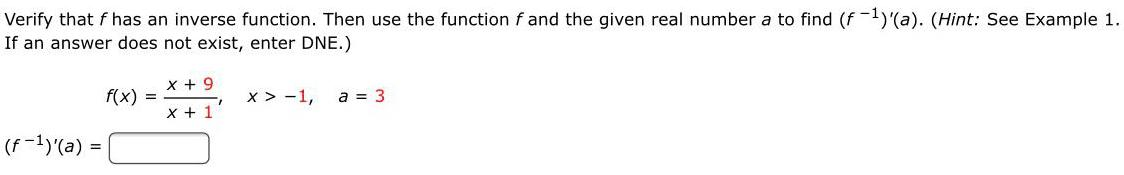Math
Differentiation
Verify that f has an inverse function. Then use the function f and the given real number a to find (f-¹)'(a). (Hint: See Example 1. If an answer does not exist, enter DNE.) f(x) =x + 9/x + 1 x > -1, a = 3 (f-¹)'(a)=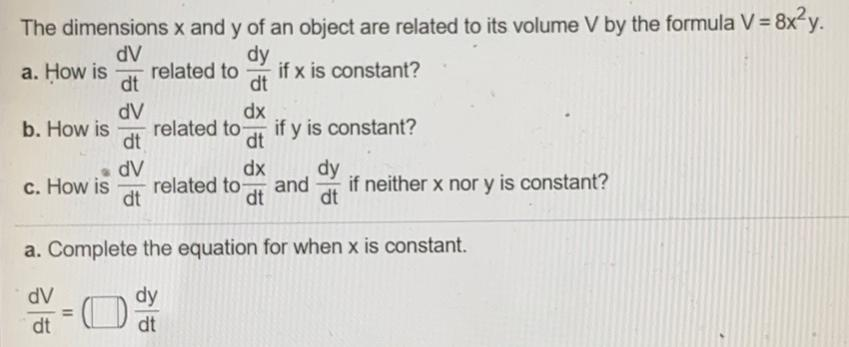Math
Differentiation
The dimensions x and y of an object are related to its volume V by the formula V = 8x²y. a. How is dV/dt related to dy/dt if x is constant? b. How is dV/dt related to dx/dt if y is constant? c. How is dV/dt related to dx/dt and dy/dt if neither x nor y is constant?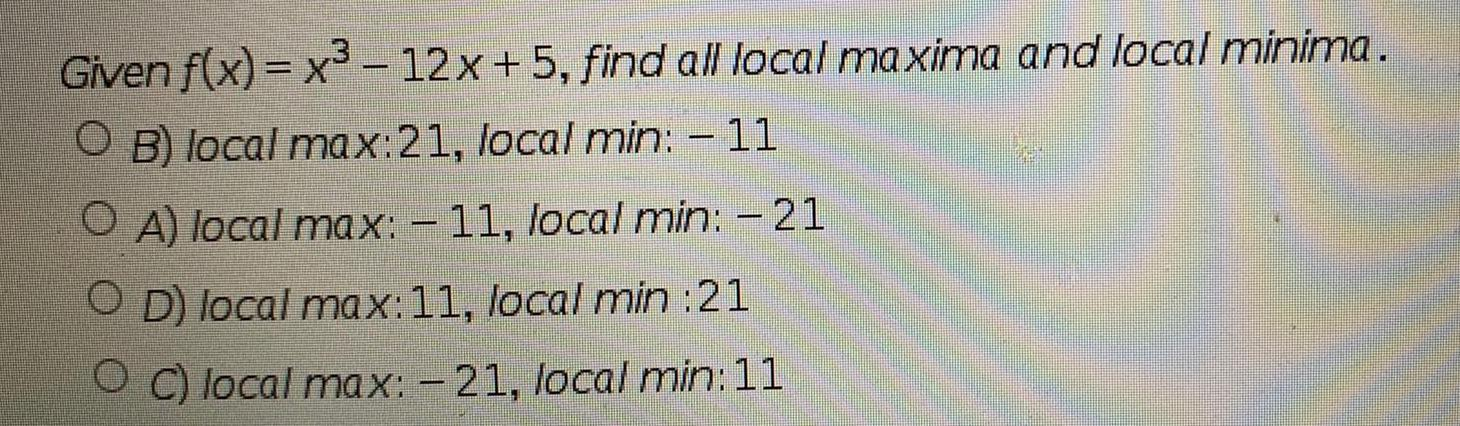Math
Differentiation
Given f(x)= x³-12x+5, find all local maxima and local minima. B) local max:21, local min: - 11 A) local max: - 11, local min: - 21 D) local max: 11, local min:21 C) local max: -21, local min:11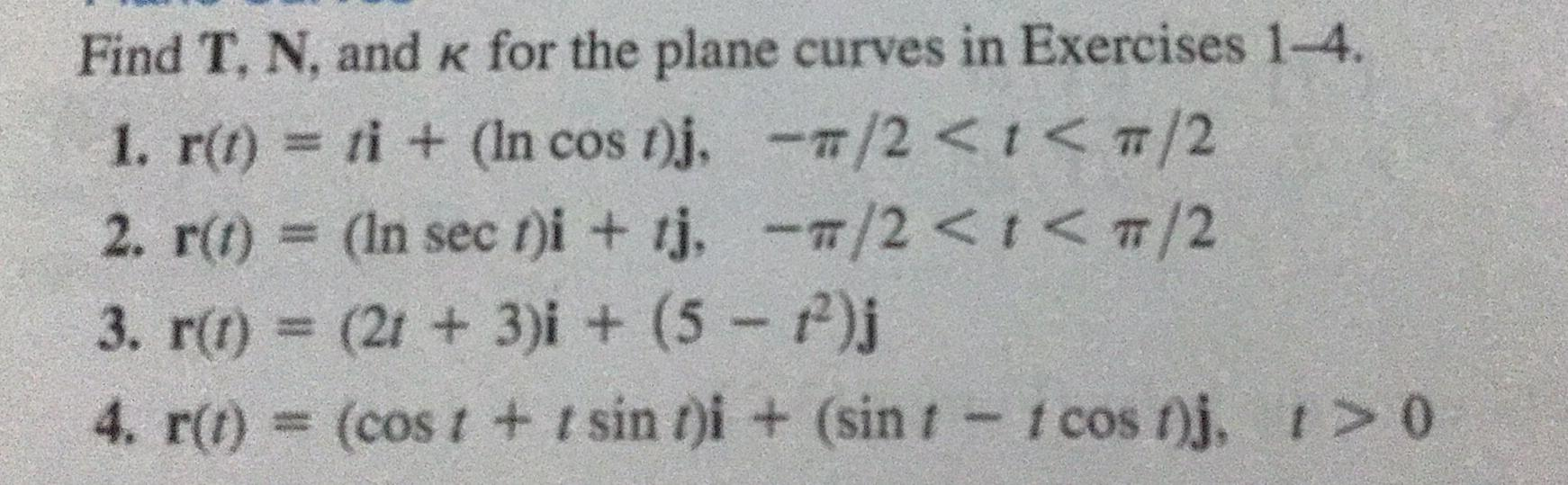Math
Differentiation
Find T, N, and K for the plane curves in Exercises 1-4. 1. r(t) = ti + (In cos t)j,-π/2 < t< π/2 2. r(t) = (In sect)i + tj,-π/2 < t< π/2 3. r(t) = (2t+ 3)i + (5 −t²)j 4. r(t) = (cost + t sin t)i + (sin 1 - 1 cost)j, t> 0